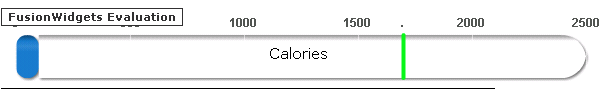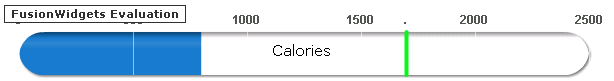## Recommended Posts

Hi,

I was working on linear gauge . And i found this weird thing. when the data is too small....the rounded cornner of the linear gauge becomes somewat flaten . It works fine with larger data.Is there a flaw in my xml .

<

Chart bgColor="FFFFFF" showTickMarks='0' showTickValues='0' showGaugeBorder='0' gaugeFillMix='{light-1},{light-1},{light-1},{light-1},{light-1},{light-1},{light-10},{light-1}, {dark-40}' gaugeFillRatio='' bgAlpha="0" majorTMHeight='10' use3DLighting='0' plotGradientColor='' tickMarkDistance='0' showBorder="0" ticksBelowGauge='0' placeTicksInside='1' placeValuesInside='0' majorTMNumber='5' minorTMNumber='0' upperLimit="125" lowerLimit="0" gaugeRoundRadius="25" chartBottomMargin="10" showGaugeLabels="1" >

<

colorRange>

<!--<color minValue='25' maxValue='80' code='#1778C9' label='Calories' alpha='0'/>-->

<color minValue="0" maxValue="5" name="" code="1677c8" />

<color minValue="5" maxValue="125" name="" code="FFFFFF" />

</colorRange>With big data